LOG RECONSTRUCTION BASICS
Good quality sonic and density log data is required for calculating

a petrophysical analysis, or the elastic properties of the rocks. Rough borehole conditions and gas effect are the most common problems that will need to be repaired.

Exactly what you do to reconstruct the log data will depend on what you want to do with that data. For example, in a conventional quantitative petrophysical analysis, we go to great lengths to avoid using bad data to obtain our results. Gas effect in the invaded zone is handled by well established mathematical techniques or by calibration of results to core analysis data if the logs are inadequate for the purpose.

For stimulation design modeling, you want the logs to accurately represent a water-filled reservoir. Since logs read the invaded zone, light hydrocarbons (light oil or gas) make the density log read too low and the sonic log read too high, compared to the water filled case. The magnitude of the error cannot be estimated without reconstructing the logs from an accurate petrophysical analysis.

The light hydrocarbon effect  problem alone would lead to erroneous elastic properties and erroneous Poisson' Ratio, Young's Modulus, and closure stress predictions. Add some rough borehole effects, and you have a meaningless set of elastic properties for stimulation modeling. Don't despair, there is a solution.

Geophysicists modeling seismic response also need good log data for creating synthetic seismograms, calibrating seismic inversion models, and for direct hydrocarbon detection models. The problem here is quite different than either the petrophysical analysis or stimulation design cases. If light hydrocarbon effect exists in the invaded zone, this must be removed and then replaced by a set of log values representing the un-invaded reservoir condition. This is the opposite of the stimulation design problem. In seismic modeling in light hydrocarbons, the density does not read low enough and the sonic does not read high enough to represent the undisturbed reservoir. Unless we fix this, reflection coefficients are too small, inversion models of Poisson's Ratio will not be calibrated, and direct hydrocarbon interpretations will be misleading.

We call this process log editing, or log repair, or log reconstruction, or log modeling . We can also create missing log curves by the same reconstruction methods. Some calibration data is required from offset wells to do this reliably. The reconstructed logs are often called synthetic logs, to distinguish them from the original measured data set.

Reconstruction techniques are not new - they have been with us since the beginning of computer aided log analysis in the early 1970's. The problem is that few people understand the need for the work or are unfamiliar with the appropriate techniques.SIMPLIFIED WORKFLOW
The concept of log reconstruction is very simple:
2. REPLACE IT WITH BETTER DATA

The workflow for log reconstruction requires a competent petrophysical analysis for shale volume, porosity, water saturation, and lithology using as little bad log data as possible. These results are then "reverse engineered" to calculate what the log "should have read" under the modeled conditions we have imposed. The parameters required will vary depending on whether the reconstruction is for a water-filled case, an invaded-zone case, or an undisturbed reservoir, but the mathematical model is identical for all three cases.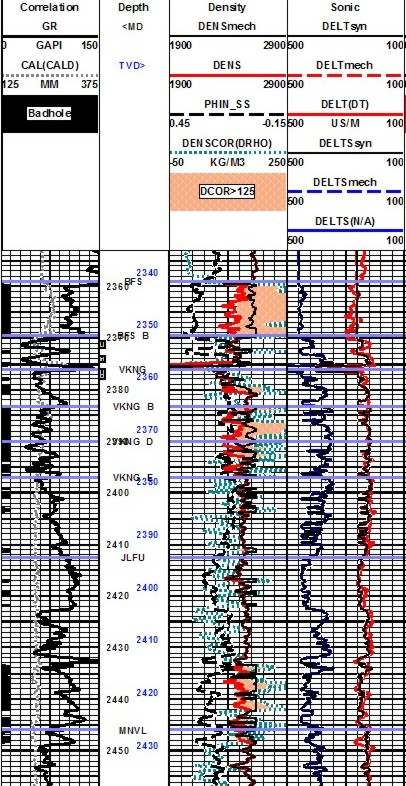In intervals where there is no bad hole or light hydrocarbon, the reconstructed logs should match the original log curves.  If it does not, some parameters in the petrophysical analysis or the reconstruction model are wrong and need to be fixed. It may take a couple of iterations. Remaining differences are then attributed to the repair of bad hole effects and light hydrocarbons in the invaded zone. It is clear from this that the reconstruction needs to encompass somewhat more than the immediate zone of interest, but not the entire borehole.

Example of synthetic density and sonic logs used to calculate elastic properties for a fracture design study. Track 1 has GR, caliper, and bad hole flag (black bar). Track 2 has density correction (dotted curve), neutron (dashed), original density (red), synthetic density (black). Track 3 shows the synthetic shear, and original and synthetic compressional sonic log curves. In this well, the sonic did not need much improvement - only small spikes were removed by the log modeling process.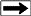There are a dozen published methods for generating synthetic logs, some dating back more than 60 years, long before the computer era. Most are too simple to do a good job, others are too complicated to be practical.

The most successful and practical model to implement and manipulate is the Log Response Equation. This equation represents the response of any single log curve to shale volume, porosity, water saturation, hydrocarbon type, and lithology.

Log editing and creation of synthetic logs is absolutely necessary in rough boreholes or when log curves are missing.

Seismic modeling, synthetic seismograms, and seismic inversion interpretations are worthless if based on bad log data.CREATING SYNTHETIC LOGS FROM THE LOG RESPONSE EQUATION
The best and easiest modern method for log reconstruction uses the Log Response Equation. Results are based on a complete and competent petrophysical analysis run using good data over the interval of interest, and little above and below that interval. This article does not cover the petrophysical analysis methods needed - they are well documented elsewhere www.spec2000.net/index.htm.

The equations needed are:
1: DENSsyn = Vsh * DENSSH + DENS1 * Vmin1 + DENS2 * Vmin2 + DENS3 * Vmin3
+ PHIe * Sw * DENSW + PHIe * (1 - Sw) * DENSHY
2: DTCsyn = Vsh * DTCSH + DTC1 * Vmin1 + DTC2 * Vmin2 + DTC3 * Vmin3
+ PHIe * Sw * DTCW + PHIe * (1 - Sw) * DTCHY

 Table 1:  KS8 – DTS / DTC Multiplier Coal 1.9 to 2.3 Shale 1.7 to 2.1 Limestone 1.8 to 1.9 Dolomite 1.7 to 1.8 Sandstone 1.6 to 1.7

3: KS8 = SUM (Vxxx * (DTS?DTCmultiplier))
4: DTSsyn = KS8 * DTCsyn

Where:
DENSsyn, DTCsyn, and DTSsyn are synthetic density, compressional and shear sonic
DENSx, DTCx, and DTSx are density and sonic parameters for each mineral and fluid (Table 2)
Vxxx = volume of each mineral present, normalized so that SUM(Vxxx) = 1.0
(DTS/DTCmultiplier) = Vp/Vs ratio for a particular mineral (Table 1)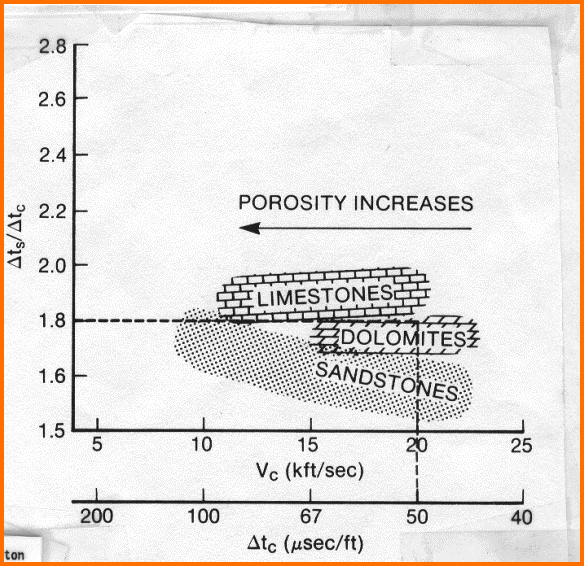NOTE: Stimulation design software wants the water filled case for its input parameters. To accomplish this, set Sw = 1.00 in equations 1 and 2, DENShy and DTChy are therefore not needed.

Equation 1 is physically rigorous. Equation 2 is the Wyllie time-average equation, which has proven exceedingly robust despite its lack of rigor. Numerical constants in Equation 3 may need some

Sharp eyed readers will notice that there is a porosity term in Equation 2, which means that Equation 4 also depends on porosity. Everyone knows that a fluid in a pore does not support a shear wave, but porosity does affect shear wave travel time in a manner similar to the compressional travel time. Consider the following equations:
5: Kc = Kp + Kb + 4/3 * N
6: DTC = 1000 / ((Kc / (0.001 * DENS)) ^ 0.5)
7: DTS = 1000 / ( (N / (0.001 * DENS)) ^ 0.5)

Bulk moduli are in GPa, density is in kg/m3, and sonic travel times are in usec/m in these equations.

It is clear from Equations 6 and 7 that both DTC and DTS depend on density, which in turn depends on mineral composition, porosity, and the type of fluid in the porosity. Both Kc and N depend on mineral composition and the presence of porosity.

Parameters used in the response equations are chosen appropriately for the case to be modeled. The Sw term varies with what you are trying to model. If you want to model the undisturbed state of the reservoir, Sw is the water saturation from a deep resistivity log and an appropriate water saturation equation. If you want to see what a log would actually read in that zone, you need the invaded zone water saturation, because that's what most logs see. Invaded zone saturation, Sxo, can be derived using a shallow resistivity curve, or it can be assumed to be Sw^(1/5).

If you want to see what a water zone would look like, Sw is set to 1.00. That is what we do for a reconstruction destined to be used in calculating rock mechanical properties for stimulation design.

In all cases, you need to select fluid parameters to match the assumptions of the model. For example, to reconstruct a log run through an invaded gas zone to reflect the undisturbed case, you need to use the undisturbed zone water saturation and appropriate fluid properties for the water and gas in each equation. Note that for stimulation design, a gas model is not required. For seismic modeling, it is required.

Matrix and fluid values for each required log curve are given in Table 1. They may need some tuning to obtain a good match to measured values. Shale values are chosen by observation of the log readings in shale intervals. You may have to look to offset wells to find a shale that does not suffer from bad hole effects.

 TABLE 2: RECOMMENDED PARAMETERS Density g/cc Density kg/m3 Compr - usec/ft Compr  usec/m Shear - usec/ft Shear  usec/m Shale 2.2 - 2.6 2200 - 2600 90 - 150 280 - 500 150 - 250 490 - 770 Water fresh 1.00 1000 200 656 Oil (light - heavy) 0.7 - 1.0 700 - 1000 250 - 188 770 - 616 Gas See Charts and Equations Below Water salt 1.10 1100 188 616 Granite 2.65 2650 55 182 80.0 262 Quartz 2.65 2650 55 182 88.8 291 Limey sandstone 2.68 2680 51 170 88.9 292 Limestone 2.71 2710 47 155 89.9 294 Limey dolomite 2.80 2800 45 150 82.3 270 Dolomite 2.87 2870 44 144 74.8 245 Anhydrite 2.90 2900 50 164 85.0 280 Coal 1.2 - 1.8 1200-1800 100 328 152+ 500+

** These represent pseudo-travel times that act as proxies in the response equations to account for the compressibility of the rock when gas, oil, or water are present. If you don't like this approach, see Biot-Gassmann method. You might like it even less. See below for more on gas and the response equation..DENSITY OF GAS FOR RESPONSE EQUATIONThe DENSsyn equation is rigorous and can be used with real hydrocarbon densities based on the temperature, pressure, and phase relationship of the fluid in question. A chart showing approximate gas density versus depth is shown at the right, based on average pressure and temperature data for the western Canadian basin.

Density of gas at reservoir conditions
- default approximationThe straight line on the graph is:
For gas, in English units  (gm/cc and feet),
6. DENSHYgas = Min (0.8, 0.000038 * DEPTH)

For gas, in Metric Units (kg/m3 and meters).
7: DENSHYgas = Min (800, 0.125 * DEPTH)

For oil, in English units (gm/cc):
8. DENSHYoil =  141.5 / (131.5 + API_GR)

For oil, in Metric  units (kg/m3):
9. DENSHYoil =  141 500 / (131.5 + API_GR)

Where:
DENSHYgas = density of gas at DEPTH
DENSHYoil = density of oil
DEPTH = depth of reservoir
API_GR = oil gravitySONIC TRAVEL TIME OF GAS FOR RESPONSE EQUATION
The DTCsyn equation, an extension of the Wyllie Time Average equation for estimating porosity in water filled rocks, provides the opportunity to compute the sonic travel time (and the seismic velocity) of any hypothetical formation by describing the quantity of rock matrix, shale, water, and hydrocarbon, as well as the acoustic properties of these elements in a given reservoir. The equation works for either compressional or shear waves, as long as the appropriate fluid and rock properties are used.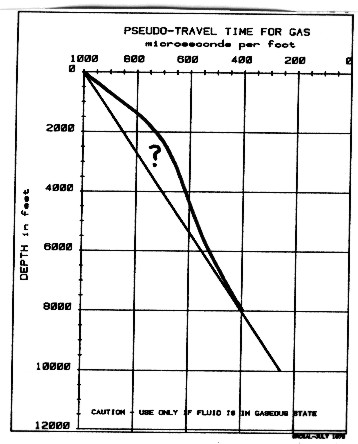Laboratory experiments and theory have shown that the time average relationship is usually not true when gas fills the pore space, or is even a small fraction of the pore space. For this reason, we call the hydrocarbon travel time in the Wyllie equation a "pseudo-travel-time" to reaffirm that it represents a velocity which may not be the same as the velocity of the gas at the temperature and pressure of the formation.

The hydrocarbon "pseudo-travel-time" is derived empirically by comparing results from synthetic seismograms and properly processed field data. A very rough approximation of hydrocarbon "pseudo-travel-time" with depth, which has given reasonable results in the western Canadian rock sequences, is shown at left. Travel time for liquids, such as oil and salt water (formation water) are more predictable and may be used in the Wyllie equation without reservation.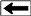Sonic travel time in gas at reservoir conditions - default approximation

This approach was first introduced by the author and John Boyd and published as "Determination of Seismic Response Using Edited Well Log Data" by E.R. Crain and J.D. Boyd at CSEG Annual Symposium, October 1979.

The straight line portion of this graph is represented by:
10: DELTHYgas = Max (200, 1000 - 0.08 * DEPTH) for English Units  (us/ft and feet)
11: DELTHYgas = Max (656, 3280 - 0.2625 * DEPTH) for Metric Units  (us/m and meters)

For oil, we have used:
12: DELTHYoil =  188 + 1.22 * API_GR       for English units
13: DELTHYoil =  616 + 4.0 * API_GR       for Metric units

Where:
DELTHYgas = compressional travel time of gas at DEPTH
DELTHYoil = compressional travel time of oil
DEPTH = depth of reservoir
API_GR = oil gravity

For shear travel time, the porosity can be accounted for by using:
11. DTSgas = DTSoil = DTSwater (see table above).META/LOG "MODL" SPREADSHEET -- Modeling Log Response
This spreadsheet models log response based on user supplied assumptions, core data, or log analysis results. It is used to prepare log data for use in Mechanical Properties of rocks or for editing logs prior to Seismic  Modeling or creation of synthetic seismic traces. The program uses the log response equation with appropriate values for fluid and rock matrix replacement.

SPR-26 META/LOG LOG RESPONSE CALCULATOR
Calculate well log response to porosity, lithology, fluid type for log editing, stimulation design, and seismic modeling.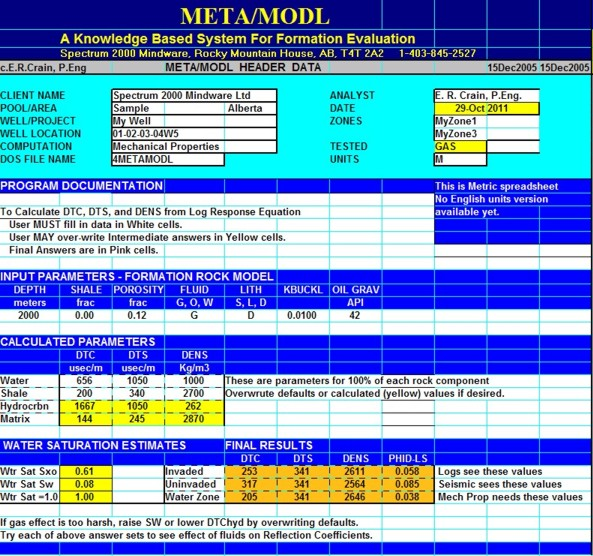Sample of META/LOG "MODL" spreadsheet for calculating log response based on user supplied assumptions,
ore data, or log analysis results.MISCELLANEOUS EDITING ROUTINES
The following algorithms may be useful in creating a shear travel time when none exists, and to quickly see the effect if gas on a sonic and density log.SHEAR TRAVELTIME FROM STONELEY WAVES DATA
In very slow formations, where shear travel time was impossible to measure on older sonic logs, this formula is used to calculate shear travel time (DTS) from Stoneley travel time {DTDT}:
14: DTSsyn = (DENS / DENSW * (DTST^2 - DTCW^2)) ^ 0.5The dipole shear sonic log has reduced the need for this calculation, as it sees shear waves better than older array sonic logs.SHEAR TRAVELTIME FROM COMPRESSIONAL DATA
A shortcut that cam be used is to determine a multiplier (Vp/Vs) based on the graph at the right:
15: DTSsyn = KS8 * DTCsyn

Where:
KS8 = 1.8 to 2.0 for shale
KS8 = 1.8 to 1.9 for limestone and anhydrite
KS8 = 1.7 to 1.8 for dolomite
KS8 = 1.6 for sandstone

Tune these parameters by comparing the synthetic shear sonic with measured shear data in an offset well.QUICKLOOK METHOD TO REMOVE GAS EFFECT
In gas zones only, the density log and the compressional sonic log data may need to be corrected to a liquid filled state. The sonic reads too high and density too low due to the gas effect. If a full blown log analysis is available, density and sonic can be back-calculated from the porosity and lithology using the response equation method described above, provided that reasonable gas corrections were made in that analysis.

In the absence of a full petrophysical analysis, the following equations will also provide better data than the raw log data. In gas zones only:
16: DENSsyn = DENS + 0.5 * PHIe * Sgxo * (DENSMA - DENSW)
17: DTCsyn = DTC - 0.5 * PHIe * Sgxo * (DTCW - DTCMA)
18: DTSsyn = DTS

Where:
DENSsyn = density corrected (gm/cc or kg/m3)
DENS = density log reading (gm/cc or kg/m3)
PHIe = effective porosity (fractional)
Sgxo = gas saturation near the well bore (fractional)  default = 0.80 for sonic, 0.70 for density log
DENSMA = matrix density (gm/cc or kg/m3)
DENSW = water density (gm/cc or kg/m3)
DTCsyn = compressional sonic corrected (usec/ft or usec/m)
DTC = compressional sonic log reading (usec/ft or usec/m)
DTCMA = compressional sonic travel time in matrix rock (usec/ft or usec/m)
DTSsyn = shear sonic corrected (usec/ft or usec/m)
DTS = shear sonic log reading (usec/ft or usec/m)
DTCW = sonic travel time in water (usec/ft or usec/m)
DTST = Stoneley travel time (usec/ft or usec/m)EXAMPLE OF LOG RECONSTRUCTION USING THE LOG RESPONSE EQUATION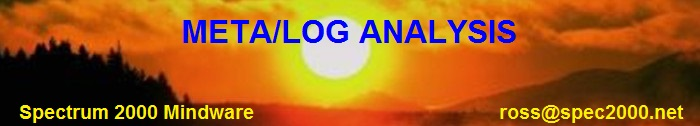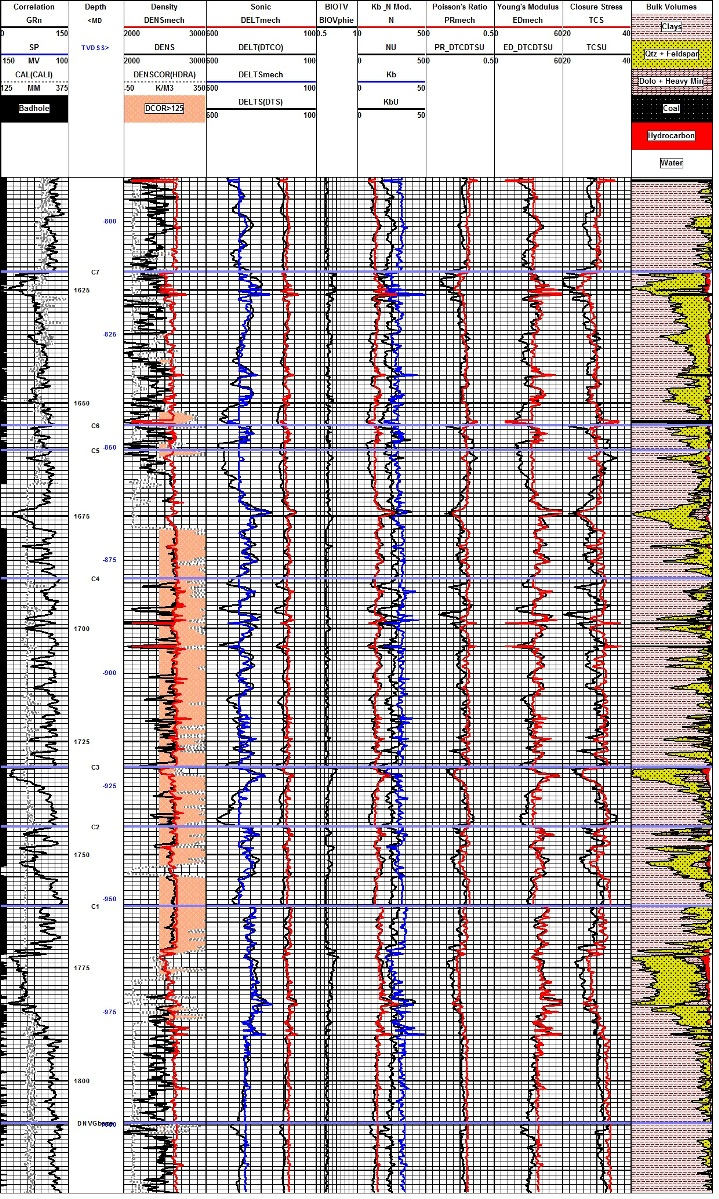Example of log reconstruction in a shaly sand sequence (Dunvegan). The 3 tracks on the left show the measured gamma ray, caliper, density, and compressional sonic. Original density and sonic are shown in black, modeled logs are in colour. Shear sonic is the model result as none was recorded in this well. Computed elastic properties are shown in the right hand tracks. Results from the original unedited curves are shown in black, those after log editing are in colour. Note that the small differences in the modeled logs compared to the original curves propagate into larger differences in the results, especially Poisson's Ratio (PR), Young's Modulus (ED), and total closure stress (TCS).

Page Views ---- Since 01 Jan 2015
Copyright 2023 by Accessible Petrophysics Ltd.
CPH Logo, "CPH", "CPH Gold Member", "CPH Platinum Member", "Crain's Rules", "Meta/Log", "Computer-Ready-Math", "Petro/Fusion Scripts" are Trademarks of the Author